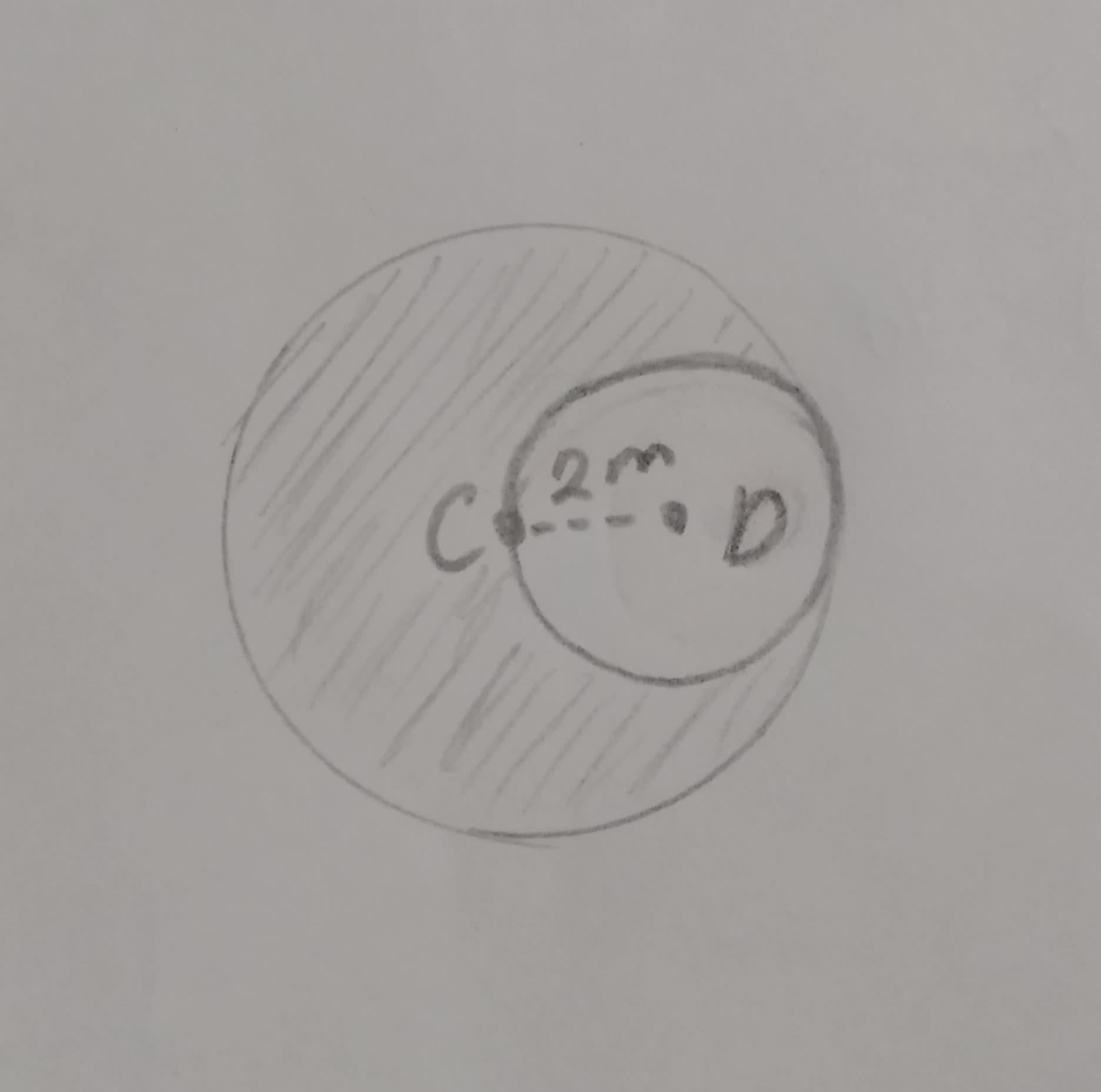# Find the area of the shaded region if C andFizeauV 2021-09-23 AnsweredFind the area of the shaded region if C and D are center

• Questions are typically answered in as fast as 30 minutes

### Plainmath recommends

• Get a detailed answer even on the hardest topics.
• Ask an expert for a step-by-step guidance to learn to do it yourself.wornoutwomanC

Given: C and D are the centers of circles radius of small circle = 2m
Radius of big circle $$= (2+2)=$$4m
Area of small circle $$\displaystyle=\pi{r}^{{2}}$$
$$\displaystyle={\frac{{{22}}}{{{7}}}}{x}{\left({2}\right)}^{{2}}$$
$$\displaystyle={\frac{{{88}}}{{{7}}}}$$ square m.
$$\displaystyle={12}\cdot{57}$$ square m.
Area of big circle $$\displaystyle={\frac{{{22}}}{{{7}}}}{x}{\left({4}\right)}^{{2}}$$
$$\frac{352}{7}$$ square m.
= Area of big circle - Area of small circle
$$\displaystyle={\frac{{{352}}}{{{7}}}}-{\frac{{{88}}}{{{7}}}}$$
$$\displaystyle={\frac{{{352}-{88}}}{{{7}}}}$$
$$\displaystyle={\frac{{{264}}}{{{7}}}}$$ square m.
$$\displaystyle\sim{37.71}$$ square m.